# Measurement Systems

Metric system (SI – international system of units): the most widely used system of measurement in the world. It is based on the basic units of meter, kilogram, second, etc.

SI common units:

 Quantity Unit Unit symbol
 Length meter m Mass (or weight) gram kg Volume litre L Time second s Temperature degree (Celsius) °C

Metric prefixes (SI prefixes):  large and small numbers are made by adding SI prefixes, which is based on multiples of 10.

Metric conversion table:

 Prefix Symbol (abbreviation) Power of 10 Multiple value Example mega M 106 1,000,000 1 Mm = 1,000,000 m kilo- k 103 1,000 1 km = 1,000 m hecto- h 102 100 1 hm = 100 m deka- da 101 10 1 dam = 10 m meter/gram/litre 1 deci- d 10-1 0.1 1 m = 10 dm centi- c 10-2 0.01 1 m = 100 cm milli- m 10-3 0.001 1 m = 1,000 mm micro µ 10-6 0.000 001 1 m = 1,000,000 µm

Metric prefix for length, weight and volume:

 Prefix Length (m – meter) Weight (g – gram) Liquid volume (L – litre) mega (M) Mm    (Megameter) Mg    (Megagram) ML     (Megalitre) kilo (k) km      (Kilometer) kg      (Kilogram) kL      (Kilolitre) hecto (h) hm      (hectometer) hg      (hectogram) hL      (hectolitre) deka (da) dam    (dekameter) dag    (dekagram) daL    (dekalitre) meter/gram/litre m        (meter) g        (gram) L        (litre) deci (d) dm      (decimeter) dg      (decigram) dL      (decilitre) centi (c) cm      (centimeter) cg      (centigram) cL      (centilitre) milli (m) mm     (millimeter) mg     (milligram) mL     (millilitre) micro (µ) µm     (micrometer) µg     (microgram) µL     (microlitre)

Steps for metric conversion:

• Identify the number of places to move the decimal point.

– Convert a smaller unit to a larger unit: move the decimal point to the left.

– Convert a larger unit to a smaller unit:  move the decimal point to the right.

Example 1.3.1

326 mm = (?) m

• Identify mm (millimeters) and m (meters) on the conversion table.

Count places from mm to m:                      3 places left• Move 3 decimal places.          (1 m = 1000 mm)

Convert a smaller unit (mm) to a larger (m) unit: move the decimal point to the left.

 326. mm = 0.326 m Move the decimal point three places to the left (326 = 326.).

Example 1.3.2

4.675 hg = (?) g

• Identify hg (hectograms) and g (grams) on the conversion table.

Count places from hg to g:                      2 places right• Move 2 decimal places.        (1 hg = 100 g)

Convert a larger unit (hg) to a smaller (g) unit:  move the decimal point to the right.

 4.765 hg = 476.5 g Move the decimal point two places to the right.

# The Unit Factor Method

Convert units using the unit factor method (or the factor-label method)

• Write the original term as a fraction (over 1).
Example:  10g can be written as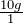• Write the conversion formula as a fraction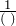or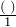.
Example:  1m = 100 cm can be written as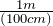or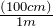• Put the desired or unknown unit on the top.
• Multiply the original term byor. (Cancel out the same units).

Example 1.3.3

1200 g = (?) kg

• Write the original term (the left side) as a fraction: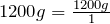• Write the conversion formula as a fraction. 1 kg = 1000 g: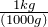• “kg” is the desired unit.
• Multiply: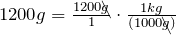The units “g” cancel out.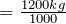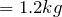Example 1.3.4

30 cm = (?) mm

• Write the original term (the left side) as a fraction: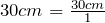• Write the conversion formula as a fraction. 1 cm = 10 mm: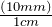“mm” is the desired unit.

• Multiply: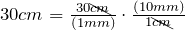The units “cm” cancel out.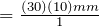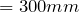Example 1.3.5

Combine after converting to the same unit.

 3 m 3000 mm 1 m = 1,000 mm –   2000 mm ——–> –   2000 mm 1000 mm
 25 kg 25000 g 1 kg = 1000 g +     4 g ——–> +      4 g 25004 g

# The Relationship between mL, g and cm3

How are mL, g, and cm3 related?• A cube takes up 1 cm3 of space (1 cm × 1 cm × 1 cm = 1cm3).
• A cube holds 1 mL of water and has a mass of 1 gram at 4°C.
• 1 cm3 = 1 mL = 1 g

Example 1.3.6

Convert.

 1) 16cm3 = ( ? ) g 16cm3 = 16 g 1 cm3 = 1 g
 2) 9 L = ( ? ) cm3 9 L = 9000 mL 1 L = 1,000 mL = 9000 cm3 1 mL = 1 cm3
 3) 35 cm3 = (?) cL 35cm3 = 35 mL 1 cm3 = 1 mL = 3.5 cL move 1 decimal place left.
 4) 450 kg = (?) L 450 kg = 450,000 g 1 kg = 1,000 g = 450,000 mL 1 g = 1 mL = 450 L 1 L = 1,000 mL

Example 1.3.7

A swimming pool measures 10 m by 8 m by 2 m. How many kilolitres of water will it hold?

 V = w l h = (8m) (10m) (2m) = 160 m3 160 m3 = ( ? ) kL 160m3 = 160,000,000 cm3 1 m = 100 cm, 3 × 2 = 6, move 6 places right for volume. 160,000,000 cm3 = 160,000,000 mL 1 mL = 1 cm3 160,000,000 mL = 160 kL 1 kL = 1,000,000 mL 160 m3 = 160 kL The swimming pool will hold 160 kL of water.

# Practice questions

1. Convert each of the following measurements:

a. 439 mm = ( ? ) m

b. 48.3 mL = ( ? ) kL

c. 7230 g = ( ? ) kg

d. 52 cm = ( ? ) mm

3. Combine:

a. 7 m – 3000 mm = ( ? ) mm

b. 63 kg + 6 g = ( ? ) g

4. Complete:

a. 38 cm3 = (       ) g

b. 5 L = (       ) cm3

c. 18 L of water has a volume of ___________ cm3 .

d. A water tank measures 45 cm by 35 cm by 25 cm. How many kilolitres of water will it hold?